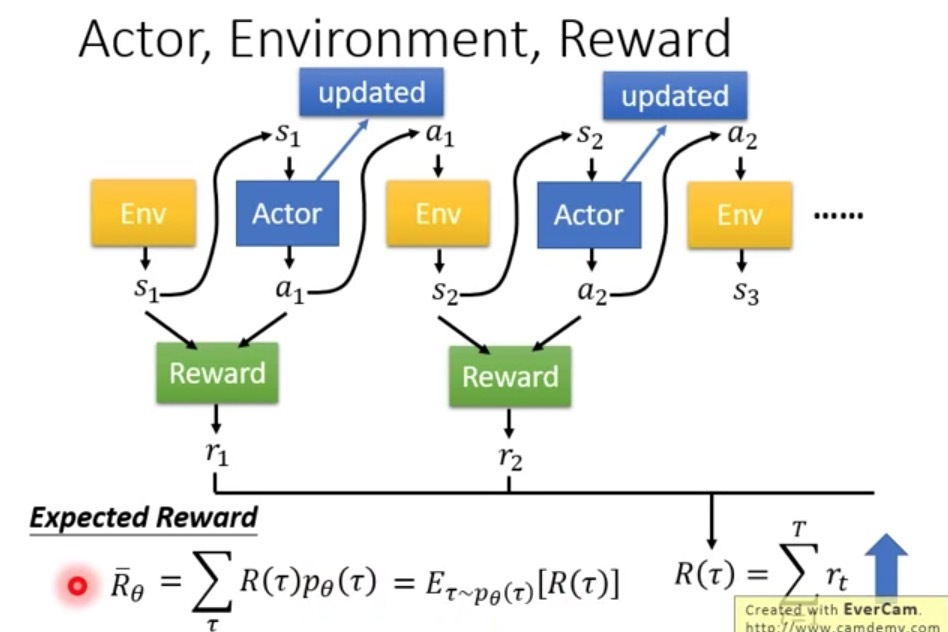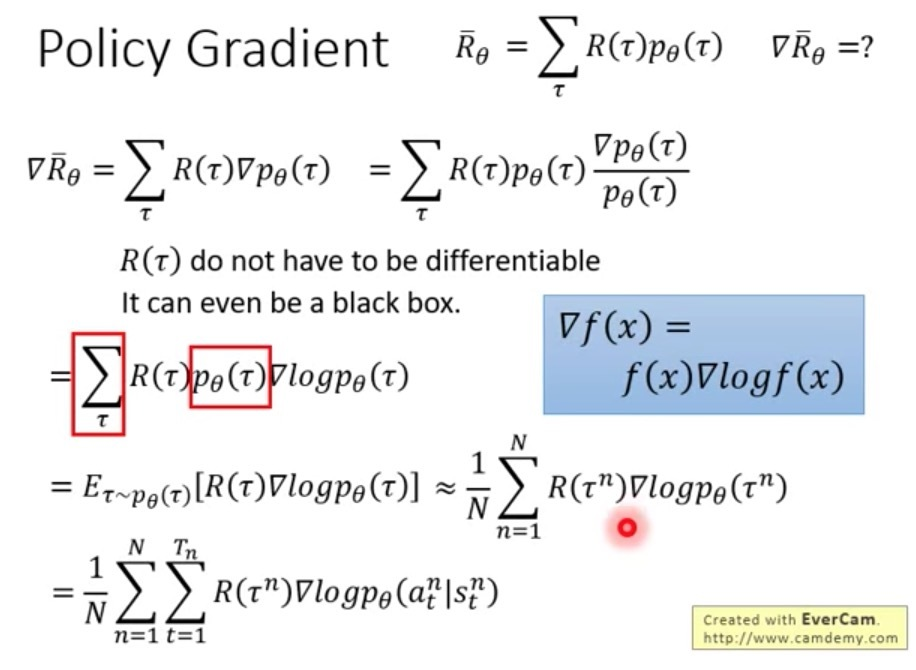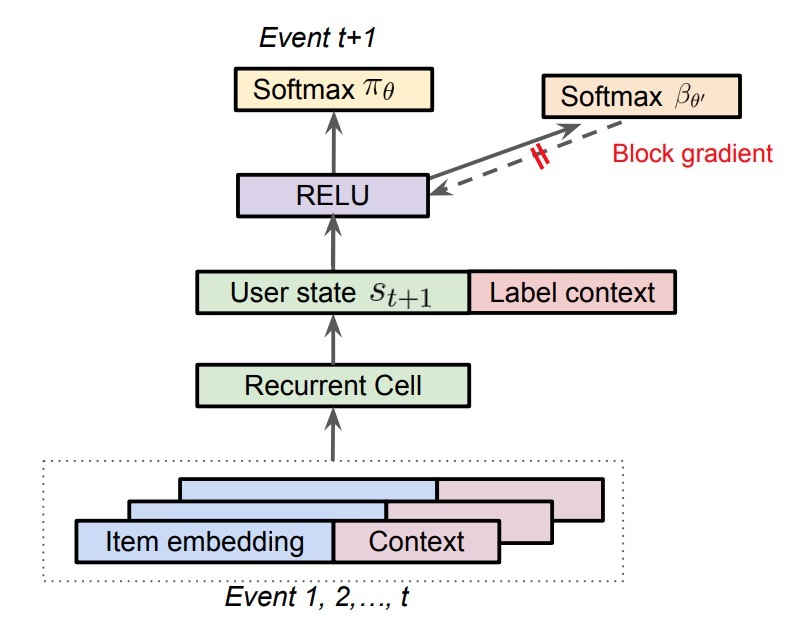## June 20, 2019

Reading time ~2 minutes

Alex Beutel等在《Top-K Off-Policy Correction for a REINFORCE Recommender System》中提出使用强化学习来提升youtube推荐。主要是从bias/variance的角度出发，具体方法如下：

# 摘要

• 1.将REINFORCE扩展到生产环境推荐系统上，动作空间有数百万；
• 2.使用off-policy correction来解决在从多种行为策略中收集的日志反馈的数据偏差（data biases）
• 3.提出了一种新的top-K off-policy correction来解释我们一次推荐多个items的策略推荐
• 4.展示了探索(exploration)的价值

# 1.介绍

• 1.REINFORCE推荐系统：我们在一个非常大的action space中，扩展了一个REINFORCE policy-gradient-based方法来学习一个神经网络推荐policy。
• 2.Off-Policy候选生成：我们使用off-policy correction来从日志反馈中学习，这些日志从之前的model policies的一个ensemble中收集而来。我们会结合一个已经学到的关于行为策略(behavior policies)的神经网络模型来纠正数据偏差。
• 3.Top-K Off-policy Correction：我们提供了一个新的top-K off-policy correction来说明：我们的推荐一次输出多个items。
• 4.真实环境的提升：我们展示了在真实环境中（在RL文献中很少有这种情况），这些方法对于提升用户长期满意度的价值。

# 3.增强推荐• S：用于描述用户状态(user states)的一个连续状态空间（state space）
• A：一个离散的动作空间(action space)，它包含了推荐可提供的items
• $P: S \times A \times S \rightarrow R$：是一个状态转移概率
• $R: S \times A \rightarrow R$：回报函数(reward function)，其中$r(s,a)$是立即回报，它会在用户状态（user state）s上执行动作a
• $\rho_0$：初始状态分布
• $\gamma$：对于future rewards的打折因子(discount factor)…(1)

…(2)

# 4.off-policy collrection

…(3)

Achiam证明了：该一阶近似对于学到的policy上的总回报的影响，会通过$O(E_{s \sim d^{\beta}} [D_{TV}(\pi \mid \beta)[s]])$来限定幅值，其中$D_{TV}$是在$\pi(\cdot \mid s)$$\beta(\cdot \mid s)$间的总方差，$d^{\beta}$是在$\beta$下的discounted future state分布。该estimator会权衡精确的off-policy correction的方差，并仍能为一个non-corrected policy gradient收集大的偏差，这更适合on-policy learning。

## 4.1 对policy $\pi_{\theta}$进行参数化

• user state （$s_t \in R^n$）: 我们对每个时间t上的user state建模，这可以同时捕获用户兴趣的演进，它使用n维向量$s_t \in R^n$来表示。
• action （$u_{a_t} \in R^m$）: 沿着该轨迹（trajectory）每个时间t上的action会使用一个m维向量$u_{a_t} \in R^m$进行嵌入。

…(4)

• $z_t \in R^n$是update gate
• $i_t \in R^n$是input gate

…(5)

• $v_a \in R^n$是每个action a在action space A中的另一个embedding
• T是时序(通常设置为1)。T值越大会在action space上产生一个更平滑的policy。

• 两个action embeddings$U \in R^{m \times \mid A \mid}$$V \in R^{n \times \mid A \mid}$
• 在RNN cell中的权重矩阵：$U_z, U_i \in R^{n \times n}, W_u, W_i, W_a \in R^{n \times m}$
• biases：$b_u, b_i \in R^n$## 4.2 估计behavior policy $\beta$

• (1) 在我们的系统中有许多agents，许多是不可控的
• (2) 一些agents具有一个deterministic policy，将$\beta$设置成0或1并不是使用这些日志反馈的最有效方式

• (1) main policy $\pi_{\theta}$会使用一个weighted softmax进行有效训练，会重点考虑长期回报(long term reward)；而behavior policy head $\beta_{\theta'}$只会使用state-action pairs进行训练
• (2) main policy head $\pi_\theta$只使用在该轨迹上具有非零回报（non-zero reward）的items进行训练；而behavior policy $\beta_{\theta'}$使用在该轨迹上的所有items进行训练，从而避免引入在$\beta$估计时的bias

## 4.3 Top-K off-policy Correction

…(6)

…(7)

• 随着$\pi_{\theta}(a\mid s) \rightarrow 0, \lambda_K(s,a) \rightarrow K$。对比起标准的off-policy correction，top-K off-policy correction会通过一个K因子来增加policy update；
• 随着$\pi_{\theta}(a \mid s) \rightarrow 1, \lambda_K(s,a) \rightarrow 0$。该乘子会使policy update归0
• 随着K的增加，以及$\pi_{\theta}(a \mid s)$会达到一个合理的范围, 该乘子会更快地将graident减小于0

## 4.4 方差减小技术

Weight Capping。

…(8)

c的值越小，会减小在梯度估计时的方差，但会引入更大的bias。

NIS(归一化重要性抽样：Normalized Importance Sampling)

TRPO(Trusted Region Policy Optimization). TRPO会阻止new policy $\pi$背离behavior policy，它通过增加一个正则项来惩罚这两个policies的KL散度。它会达到与weight capping相似的效果。

。。。

# 参考

Updated on

### BERT4Rec介绍

# 介绍从历史行为中建模用户的动态偏好，对于推荐系统来说是个挑战。之前的方法采用序列神经网络以从左到右的方式将用户历史交互编码成隐表示，来生成推荐。尽管它们是有效的，这种从左到右的单向模型是次优的，我们对此仍有争论，因为有以下的限制：- a) 单向结构限制了在用户行为序列中...… Continue reading

#### DSIN介绍

Published on May 27, 2019

#### MIND召回介绍

Published on May 25, 2019